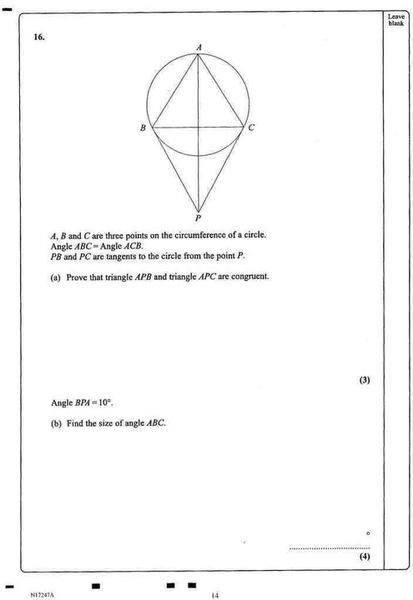# Congruent Triangle in circle

• thomas49th

#### thomas49thSorry, it's upside down :(

How do i go about solving A. I know that for somthing to be congruent it needs to

SSS
RHS
AAS
SAS

I know that C = B because they lie on a circuference and a chord that binds them is A. Where do I go from now?

Thx

thomas49th said:
I know that for somthing to be congruent it needs to

SSS
RHS
AAS
SAS

What does this mean?

abreivations of triangle congruency

take a look:
http://www.bbc.co.uk/schools/gcsebitesize/maths/shapeh/congruencyandsimilarityrev2.shtml [Broken]

Last edited by a moderator:
Angles pcb and pbc are also equal (alternate angle theorem). Therefore, pc=pb (sides opp equal angles). This gives you two sides equal and 1 side common so sss congruency is established.

I don't see how it's alternate segment theory...

The angle between a tangent and a chord is equal to the angle made by that chord in the alternate segment.

I can't see it. Do you know a good technique for spotting it?

Thx

If you extend pc to some point, say q. Then angle acq is equal to angle abc. Apply the same thing on the other side of the quad.

OKay, this is my thinking
statement 1: AP=AP Reason: common line (S)
statement 2: <ABC = <ACB reason: given
statement 3: <PBC = <PCB reason: tangent from the same point P
statement 4: therefore <ABP = <ACP reason: see statement 2&3 (A)
statement 5: <CPA = <BPA reason: tangent cords are from the same point P (A)
statement 6: triangle ABP = triangle ACP reason: AAS

but I'm not sure for statement 5, as the diagram doesn't indicate anything...